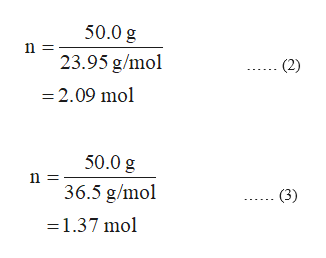# A solution containing 50.0g of LiOH is mixed with a solution containing 50.0g of HCl: (LiOH + HCl to LiCl + H2O). Which compound is in excess and what mass of LiCl is produced?

Question

A solution containing 50.0g of LiOH is mixed with a solution containing 50.0g of HCl: (LiOH + HCl to LiCl + H2O). Which compound is in excess and what mass of LiCl is produced?

check_circle

star
star
star
star
star
1 Rating
Step 1

The number of moles of a species present in the reaction is given by the expression given in the equation (1) in which n represents the number of moles, m represents the given mass of species and M represents the molar mass of species.

The mass of product formed in the reaction is calculated by considering the number of moles of limiting reagent.

Step 2

The number of moles of LiOH present in the reaction can be calculated by substituting mass and molar mass of LiOH in the equation (1) which is shown in equation (2).

The number of moles of HCl present in the given reaction is calculated by substituting mass and molar mass of HCl in the equation (2) which is shown in equation (3).

As equal number of moles of LiOH and HCl are required in the reaction but the number of moles of LiOH present in larger quantity as compared to that of HCl, therefore, LiOH is an excess reagent in the reaction.help_outlineImage Transcriptionclose50.0 g 23.95 g/mol (2) -2.09 mol 50.0 g n 36.5 g/mol (3) =1.37 mol fullscreen
Step 3

The number of moles of LiCl formed in the reaction is equal to the moles of HCl (limiting reagent) present in the reaction because the reaction will cease after the consump...

### Want to see the full answer?

See Solution

#### Want to see this answer and more?

Solutions are written by subject experts who are available 24/7. Questions are typically answered within 1 hour.*

See Solution
*Response times may vary by subject and question.
Tagged in

### Other